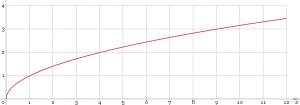# How to Find the Square Root Using Binary Search

Are you eager to know how to find the square root using binary search? Be quick to examine our example below. The lucid explanation of the issue was prepared by an expert from our service. All of our experts, including the one who completed this sample, are well-educated and knowledgeable in a variety of areas. AssignmentShark is a service that helps students with their technical tasks. This means that if you are having difficulties with math, physics, IT, statistics, biology, or chemistry, you have an opportunity to receive assistance from us.

Our blog is filled with samples completed by experts that you can use for solving your own tasks. Also, you can ask our expert to complete a specific sample just for you. The only thing you need to do is to specify your requirements. No matter whether you are a high school, college, or university student, we can provide you with help. Be quick to investigate our sample on how to find the square root using binary search and decide whether you need more online assignment help!

## Using Binary Search to Find the Square Root

We need to implement a method of finding the square root without using the available functions of exponentiation and root extraction.Solution

From school mathematics we remember that the square root function is increasing throughout its region of definition (if you don’t remember or it’s not obvious, you can take the derivative and make sure it is greater than zero), which means we can use the algorithm of binary search.

Let’s assume we need to extract the square root from the number x. So we set the left border of the binary search on 0 and the right on max(1, x). In this condition we will consider all possible cases: on the interval 0..1 the root of a number is greater than the number, and in the interval 1..inf — less than the number.

Now let’s find the arithmetic mean of the boundaries, call it m, and check out if m * m is bigger than x. If so, the desired answer is on the number line to the left of m, and therefore, it is necessary to make m the right border of the binary search, otherwise — left. After a few hundred iterations, we will find the square root by satisfying all the needs of accuracy.

So here’s the implementation of the function which will find the square root using C++:

#include <math.h>

#include <time.h>

#include <stdlib.h>

#include <sys/time.h>

#include <iostream>

#define E 1e-4

float findSqrt(float x){

if(x < 0){

std::cout << “Error in the input x” << std::endl;

return -1;

}

float a = 1;

float b = x;

if(x < 1){

a = x;

b = 1;

}

float m = (a + b) / 2;

float error = m * m – x;

while(fabs(error) > E){

4 if(error > 0){

b = m;

}else{

a = m;

}

m = (a + b) / 2;

error = m * m – x;

}

return m;

}